# Divide

There's no DIVIDE function in Excel. Simply use the forward slash (/) to divide numbers in Excel.

1. The formula below divides numbers in a cell. Use the forward slash (/) as the division operator. Don't forget, always start a formula with an equal sign (=).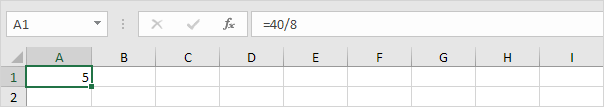2. The formula below divides the value in cell A1 by the value in cell B1.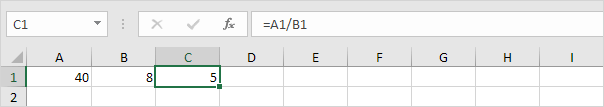3. Excel displays the #DIV/0! error when a formula tries to divide a number by 0 or an empty cell.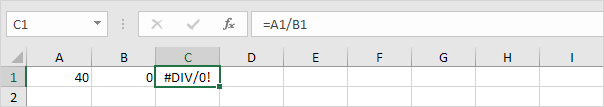4. The formula below divides 43 by 8. Nothing special.5. You can use the QUOTIENT function in Excel to return the integer portion of a division. This function discards the remainder of a division.6. The MOD function in Excel returns the remainder of a division.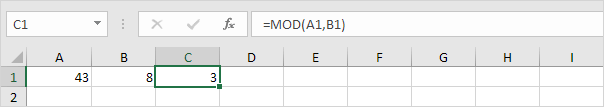Take a look at the screenshot below. To divide the numbers in one column by the numbers in another column, execute the following steps.

7a. First, divide the value in cell A1 by the value in cell B1.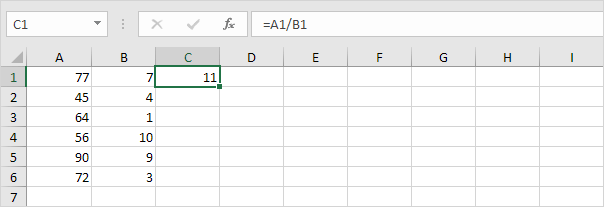7b. Next, select cell C1, click on the lower right corner of cell C1 and drag it down to cell C6.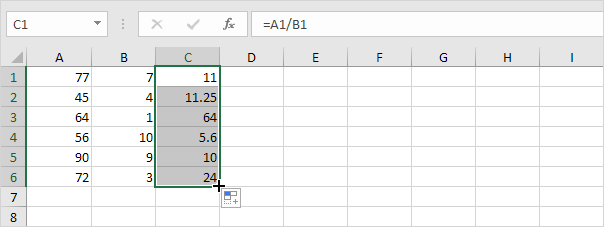Take a look at the screenshot below. To divide a column of numbers by a constant number, execute the following steps.

8a. First, divide the value in cell A1 by the value in cell A8. Fix the reference to cell A8 by placing a \$ symbol in front of the column letter and row number (\$A\$8).8b. Next, select cell B1, click on the lower right corner of cell B1 and drag it down to cell B6.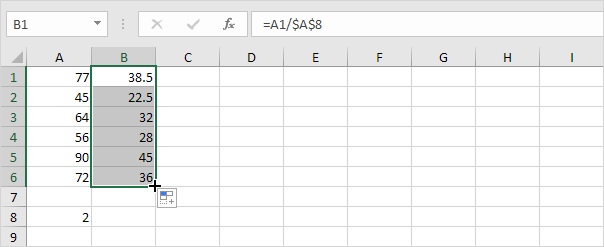Explanation: when we drag the formula down, the absolute reference (\$A\$8) stays the same, while the relative reference (A1) changes to A2, A3, A4, etc.

If you're not a formula hero, use Paste Special to divide in Excel without using formulas!

9. For example, select cell C1.10. Right click, and then click Copy (or press CTRL + c).

11. Select the range A1:A6.

12. Right click, and then click Paste Special.

13. Click Divide.14. Click OK.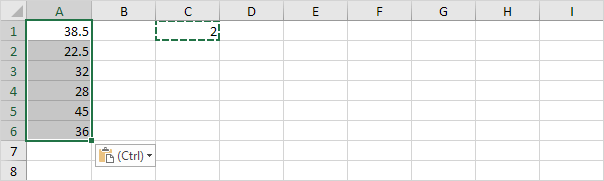Note: to divide numbers in one column by numbers in another column, at step 9, simply select a range instead of a cell.

Go to Next Chapter: Ribbon